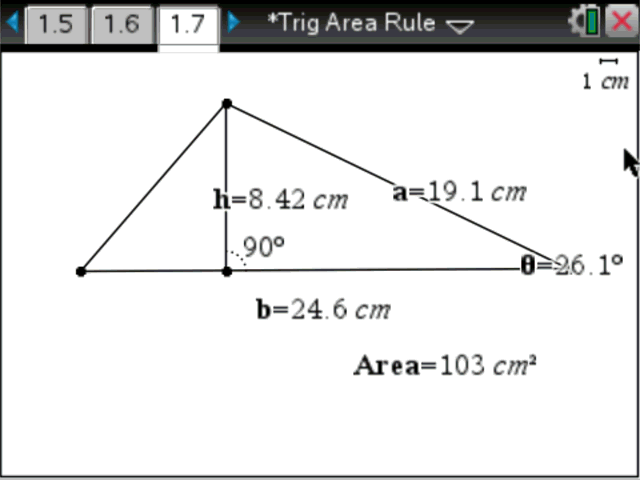Activities

•• Subject Area

• Mathematics: Measurement: Measurement
• Standard: National Curriculum 7-10: Measurement and Geometry: Shape

• AuthorMiddle
Aust Senior

50 Minutes

• Device
• TI-Nspire™
• TI-Nspire™ CAS
• TI-Nspire™ Navigator™
• Software

TI-Nspire™
TI-Nspire™ CAS
TI-Nspire™ CAS Navigator™ NC System
TI-Nspire™ Navigator™ NC System

3.2

Jack’s Tile Problem – Exploring the Area Rule for TrianglesActivity Overview

In this activity, students change the shape of a triangle and capture the dimensions into a spreadsheet. They then explore and compare the two rules for the area of a triangle: Area = 1/2.b.h and Area = 1/2.a.b.sin(C)

Objectives

The objective of this activity is to show students how the area of a triangle formula Area = 1/2.a.b.sin(C) can be used to find the area of non-right angle triangles.

Vocabulary

• Area
• Triangle
• Non-right angle